International
Tables for
Crystallography
Volume F
Crystallography of biological macromolecules
Edited by E. Arnold, D. M. Himmel and M. G. Rossmann

International Tables for Crystallography (2012). Vol. F, ch. 11.4, pp. 288-289   | 1 | 2 |

Section 11.4.6. Prediction of the diffraction pattern

Z. Otwinowski,a* W. Minor,b D. Boreka and M. Cymborowskib

aUT Southwestern Medical Center at Dallas, 5323 Harry Hines Boulevard, Dallas, TX 75390–9038, USA, and bDepartment of Molecular Physiology and Biological Physics, University of Virginia, 1300 Jefferson Park Avenue, Charlottesville, VA 22908, USA
Correspondence e-mail:  zbyszek@work.swmed.edu

11.4.6. Prediction of the diffraction pattern

| top | pdf |

The autoindexing procedure assigns Miller indices only to strong spots, ones that can be found through a peak-search procedure. The target of the experiment is to estimate structure-factor amplitudes for all reflections captured by the detector. Therefore, positions of all spots need to be predicted by applying the following equations to all possible triplets h. Using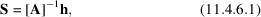a matrix [A] must be found that generates the vector S that satisfies the diffraction condition [equation (11.4.2.1)], knowing that the matrix [A] is a function of the crystal orientation at the goniostat angles that generated the reflection with indices h [equation (11.4.2.6)]. The rotation of the crystal during the experiment creates a straightforward algebraic problem that results in a complex equation defining the angle at which the reflection occurs. This angle consequently defines the image or images on which the reflection appears. Knowing this angle, the vector S can be calculated, and, from equation (11.4.2.5), the direction of the vector X can be found:Calculation of the length of vector X requires a knowledge of detector orientation, which, for flat detectors, is described here by vector G, perpendicular to the detector and with length equal to the crystal-to-detector distance: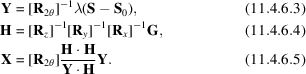Then, by inverting equation (11.4.2.8), the position in pixelsof the reflection can be calculated: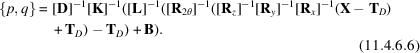11.4.6.1. Refinement of crystal and detector parameters

| top | pdf |

The precision of the integration step depends on precise knowledge of the peak positions. The autoindexing step provides only an approximate orientation of the crystal, and the result of that step is imprecise if the initial values of the detector parameters are poorly known. A nonlinear least-squares refinement process is used to improve the prediction (EEC Cooperative Workshop on Position-Sensitive Detector Software, 1986). Depending on the particulars of the experiment, the same parameters (e.g. crystal-to-detector distance) may either be known more precisely a priori, or are better estimated from the diffraction data. DENZO allows either fixing or refining of each of the parameters separately. This flexibility is important when characterizing a detector, but when detector parameters are already known, the fit all option and detector-specific default values are quite reliable.

DENZO can refine the six parameters describing the position and orientation of the detector in space. It can also refine internal parameters of the detector including:

 (1) curvature radius and rotation of the active surface – film rotation, for cylindrical detectors; (2) x to y scale and x to y skew; (3) radial/angular distortion for spiral scanners; and (4) polynomial distortion, separate for each CCD module of multi-CCD detectors (Naday et al., 1998).

Detector- and crystal-parameter refinement in DENZO is achieved by minimizing the sum of three functions [equations (11.4.6.7), (11.4.6.8)and (11.4.6.11)] of the type in equation (11.4.5.1). The contribution resulting from the measurement of position {p, q} of the reflection is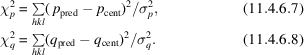where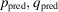is the predicted position of the reflection in pixel coordinates,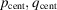is the centroid position of the observed reflection in pixel coordinates and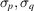are combined estimates of uncertainties of the observed and predicted positions.

11.4.6.2. Bragg's law for non-ideal conditions: mosaicity

| top | pdf |

The Bragg condition [equation (11.4.2.1)] assumes diffraction from ideal crystals and a parallel X-ray beam. In reality, crystals are mosaic and the beam has some angular spread. The value of the mosaicity keyword describes the range of orientations of the crystal lattice within a sample. As the impacts of mosaicity and the beam's angular spread on the angular width of reflections are equivalent, the keyword mosaicity describes the sum of both effects.

DENZO assumes the following model of angular shape of diffraction peaks for the oscillation angle: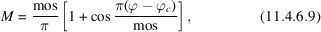where mos is the observed angular width of a diffraction peak yet to be increased by the Lorentz factor,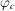is the predicted oscillation angle at which the diffraction condition is fulfilled,is in the range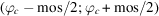, otherwise M = 0. The actual width of the reflection is different due to Lorentz-factor variability over the image, so equation (11.4.6.9)describes only the common component of the angular width.

Using equation (11.4.6.9)we can calculate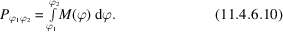P is the predicted partiality of data collected by oscillating fromto. It is a number that represents what fraction of the reflection intensity is present in one image. If the partiality is 1, such reflections are called fully recorded; otherwise, they are called partials. For partials, predictions of partiality can be compared with the observed fractionof the reflection intensity present in one image. The partiality model contributes the following term to the refinement: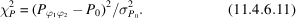The combined positional [described by equations (11.4.6.7)and (11.4.6.8)] and partiality refinement [equation (11.4.6.11)] used in DENZO is both stable and very accurate. The power of this method lies in proper weighting by estimated errors of two very different terms – one describing positional differences and the other describing intensity differences. Both detector and crystal variables are uniformly treated in the refinement process.

11.4.6.3. Detector distortions

| top | pdf |

The design of detectors results in pixels not being positioned on an exact square or rectangular grid. A correct understanding of the detector distortions is essential to accurate positional refinement. The types of distortions are detector-specific. The primary sources of error include misalignment of the detector position sensors and optical or magnetic distortion in CCD-based detectors. If the detector distortion can be parameterized, then these parameters should be added to the refinement. For example, in the case of spiral scanners, there are two parameters describing the end position of the scanning head. In a perfectly adjusted scanner, these parameters would be zero. In practice, however, they may deviate from zero by as much as 1 mm. Such misalignment parameters can correlate very strongly with other detector and crystal parameters, particularly for low-symmetry lattices or for low-resolution data. If the distortions are stable, it is better to determine them in a separate experiment optimized for that task.

Fibre-optic tapers used in many CCD detectors have distortions that have to be individually determined for each instrument. The distortion is stable over time and its spatial characteristics are dominated by a smooth component and a small local shear. In high-quality tapers used in X-ray instruments, the small local shear can be ignored. The smooth component can be parameterized in a number of ways, for example by splines or polynomials (Messerschmidt & Pflugrath, 1987). DENZO uses two-dimensional Chebyschev polynomials (Press et al., 1989) in {x, y} or {p, q} coordinates, normalized to the range,. Typically, fifth- or seventh-order polynomials result in a positional error (r.m.s.) lower than 7 µm, which is about one tenth of a typical detector pixel. DENZO can use either a grid mask pattern or the X-ray diffraction pattern to refine the coefficients of the Chebyschev polynomials. If a grid mask is used, it has to be precisely made and positioned. The use of crystallographic data requires precise knowledge of detector and crystal parameters that are not known a priori with the required precision. The crystal and detector parameters can be determined in the same experiment as detector distortion. However, this experiment needs to be designed to minimize the impact of correlations between the parameters involved. The data analysis requires the description of the distortion function and its inverse. In DENZO, both are approximated in terms of Chebyschev polynomials. The magnitude of the approximation error is the same for the distortion function and its inverse.

References

EEC Cooperative Workshop on Position-Sensitive Detector Software (1986). Phase I and II, LURE, Paris, 16 May – 7 June; Phase III, LURE, Paris, 12–19 November.
Messerschmidt, A. & Pflugrath, J. W. (1987). Crystal orientation and X-ray pattern prediction routines for area-detector diffraction systems in macromolecular crystallography. J. Appl. Cryst. 20, 306–315.
Naday, I., Ross, S., Westbrook, E. M. & Zentai, G. (1998). Charge-coupled device/fiber optic taper array X-ray detector for protein crystallography. Opt. Eng. 37, 1235–1244.
Press, W. H., Flannery, B. P., Teukolsky, S. A. & Vetterling, W. T. (1989). Numerical Recipes – the Art of Scientific Computing. Cambridge University Press.